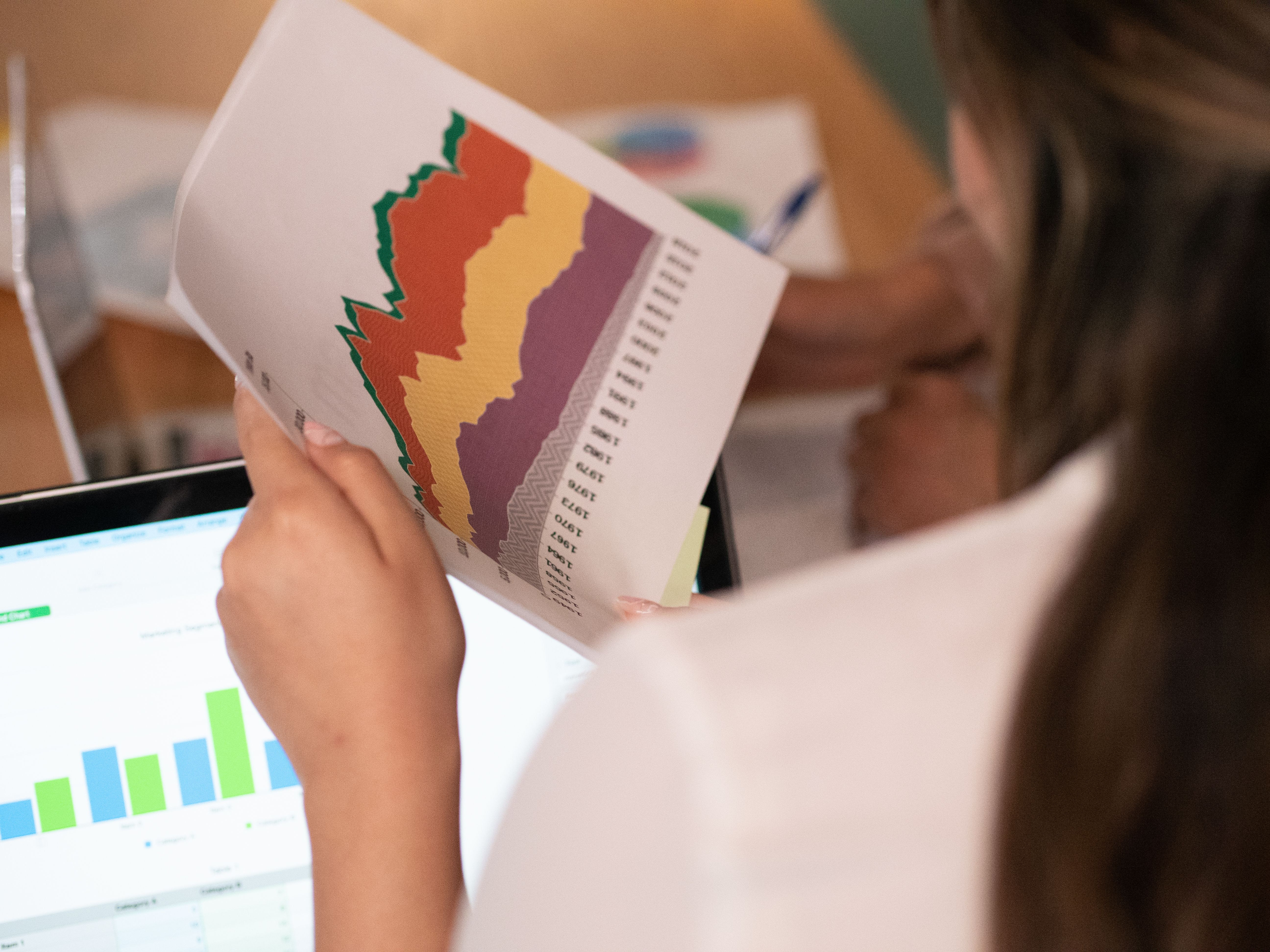# What is high school elementary statistics?

High School Elementary Statistics

# High School Elementary Statistics## What is High School Elementary Statistics?

High School Elementary Statistics, also called Elementary Statistics (11-12), is a course that covers basic concepts of statistics and probability, which are applicable to all disciplines. The topics covered include data analysis, probability, estimation, statistical hypotheses, regression, and correlation. This course introduces students to the statistical methods used across different fields, preparing them for advanced courses in math, science, economics, or social sciences.

## FAQ

### What is Data Analysis?

Data Analysis is the process of collecting, organizing, interpreting, and presenting data. It involves the use of statistical methods to extract meaning from data and draw conclusions from it. In High School Elementary Statistics, students learn to use descriptive statistics, such as mean, median, mode, range, and standard deviation, to summarize data and make sense of it. They also learn to use graphs, tables, and charts, such as histograms and scatterplots, to visualize the data and identify patterns, trends, and outliers.In addition to descriptive statistics, High School Elementary Statistics covers inferential statistics, which aims to make predictions or draw conclusions about a population based on a sample of data. Students learn to use probability theory and sampling techniques to estimate population parameters, such as population mean and proportion, and to test statistical hypotheses, such as whether two populations are different or whether a correlation exists between two variables. These techniques are widely used in scientific research, market analysis, public opinion polls, and other fields where data-driven decisions are made.

### What is Probability?

Probability is the branch of mathematics that deals with the study of random events, such as coin tosses, dice rolls, or weather forecasts, where the outcome cannot be predicted with certainty. In High School Elementary Statistics, students learn the basics of probability theory, such as the rules of counting, the notion of independent and dependent events, and the concept of probability distributions. They also learn to apply probability theory to solve problems that involve sampling, experiments, or games of chance.Probability theory is used in countless fields, such as physics, engineering, finance, biology, and computer science, among others. It provides a framework for modeling uncertain events and making informed decisions based on data. For example, medical researchers may use probability to estimate the effectiveness of a new drug, financial analysts may use probability to calculate the risk of an investment, and meteorologists may use probability to predict the likelihood of a tornado.

### What is Regression and Correlation?

Regression and Correlation are two closely related statistical techniques that are used to analyze the relationship between two variables. In High School Elementary Statistics, students learn to use regression analysis to model the linear relationship between a response variable (dependent variable) and one or more predictor variables (independent variables). They also learn to use correlation analysis to measure the strength and direction of the association between two quantitative variables.Regression and correlation analysis are widely used in social sciences, economics, psychology, and other fields where researchers seek to understand the causal relationship between two or more variables. For example, a sociologist may use regression analysis to examine the effect of education on income, a marketer may use regression analysis to predict the sales of a product based on its price and advertising, and a psychologist may use correlation analysis to investigate the relationship between stress and health.